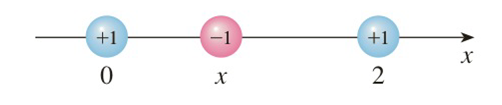Chapter 3.5, Problem 44E

Chapter
Section
Textbook Problem

# Coulomb’s Law states that the force of attraction between two charged particles is directly proportional to the product of the charges and inversely proportional to the square of the distance between them. The figure shows particles with charge 1 located at positions 0 and 2 on a coordinate line and a particle with charge − 1 at a position x between them. It follows from Coulomb’s Law that the net force acting on the middle particle is F ( x ) = − k x 2 + k ( x − 2 ) 2       0<x<2 where k is a positive constant. Sketch the graph of the net force function. What does the graph say about the force?To determine

To sketch:

The curve of y

Explanation

1) Concept:

i) The domain is the set of x values for which the function is defined.

ii) To find x-intercept, put y=0, and to find y-intercept, put x=0 in the given function.

iii) Symmetry: To find symmetry, replace x by –x and check the behaviour of function. Thus, if f-x=fx, then it is an even function, so its graph is symmetric about the y-axis. If f-x=-fx, then it is an odd function, so its graph is symmetric about the origin. And if f-x-fxfx, then it has no symmetry.

iv) An asymptote is a tangent at infinity. To find horizontal, vertical, and slant asymptote, follow the rules.

v) A function is increasing if f'x>0  and decreasing if f'x<0 in that particular interval.

vi) The number f(c) is a local maximum value of f  if fcf(x) when x is near c and is a local minimum value of f if fc f(x) when x is near c.

vii) If f''x>0, the function is concave up and if f''x<0, the function is concave down in that particular interval. And f''x=0, gives the values of inflection points

2) Given:

Fx=-kx2+kx-22, k>0, 0<x<2

3) Calculations:

Here, first find the domain of the given function and the x & y intercepts. Next, check the symmetry, asymptotes, intervals of increase and decrease, local maximum and minimum values, concavity, and points of inflection. Using these, sketch the curve

A. Domain

The domain is (0, 2).

B. Intercepts:

There is no y intercept

For x intercept, put y=0 in the given equation

0=-kx2+kx-22

kx2=kx-22

x-22=x2

-4x+4=0

x=1

So x intercept is 1, 0

C. Asymptote

a) Horizontal asymptotes

There is no horizontal asymptote

b) Vertical asymptotes

limx2--kx2+kx-22=

limx0+-kx2+kx-22=

So there are vertical asymptotes at x=0, 2

D

### Still sussing out bartleby?

Check out a sample textbook solution.

See a sample solution

#### The Solution to Your Study Problems

Bartleby provides explanations to thousands of textbook problems written by our experts, many with advanced degrees!

Get Started

#### Find more solutions based on key concepts